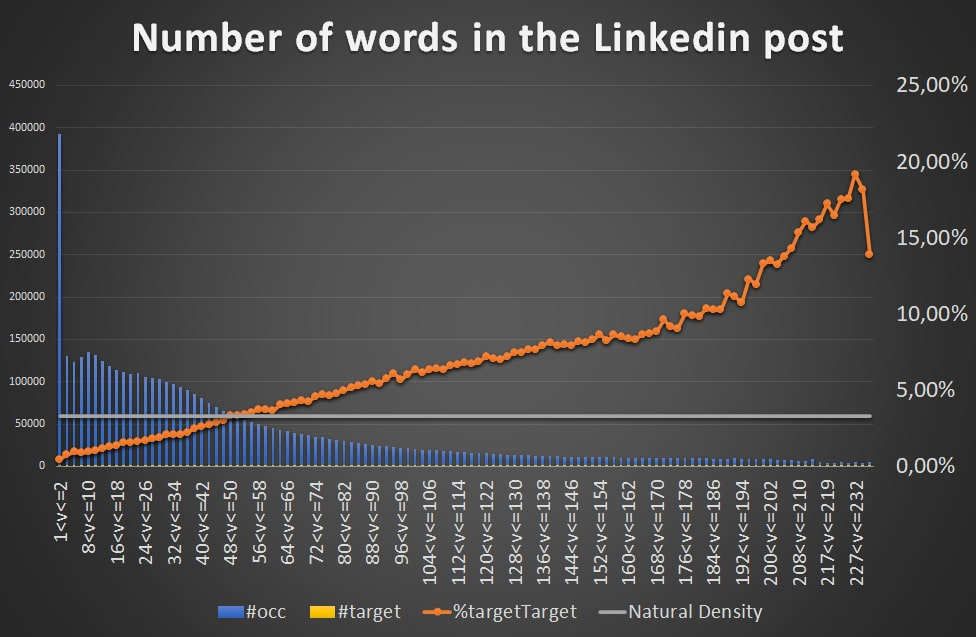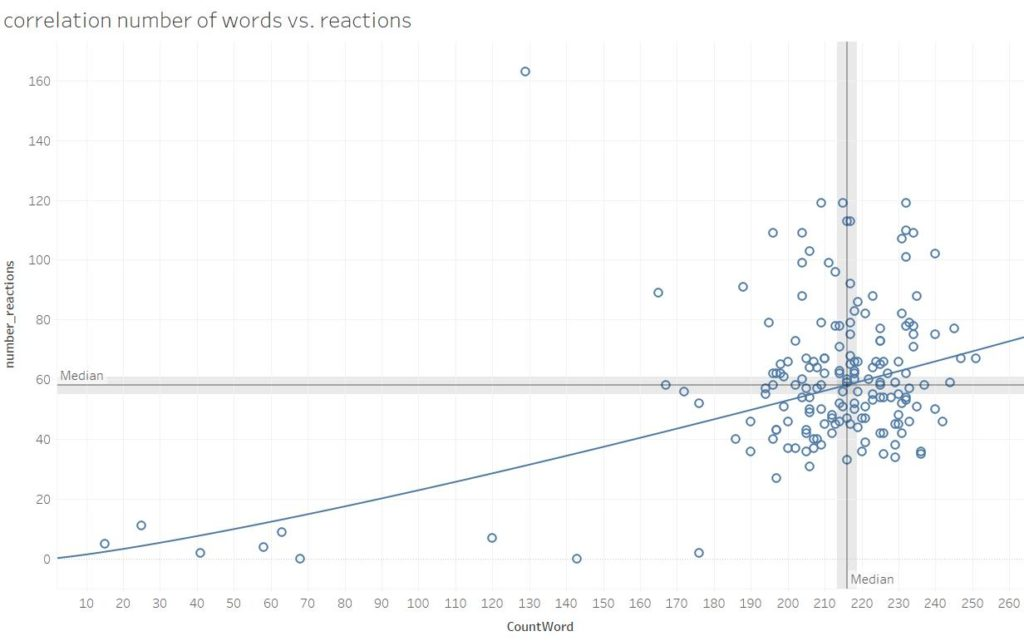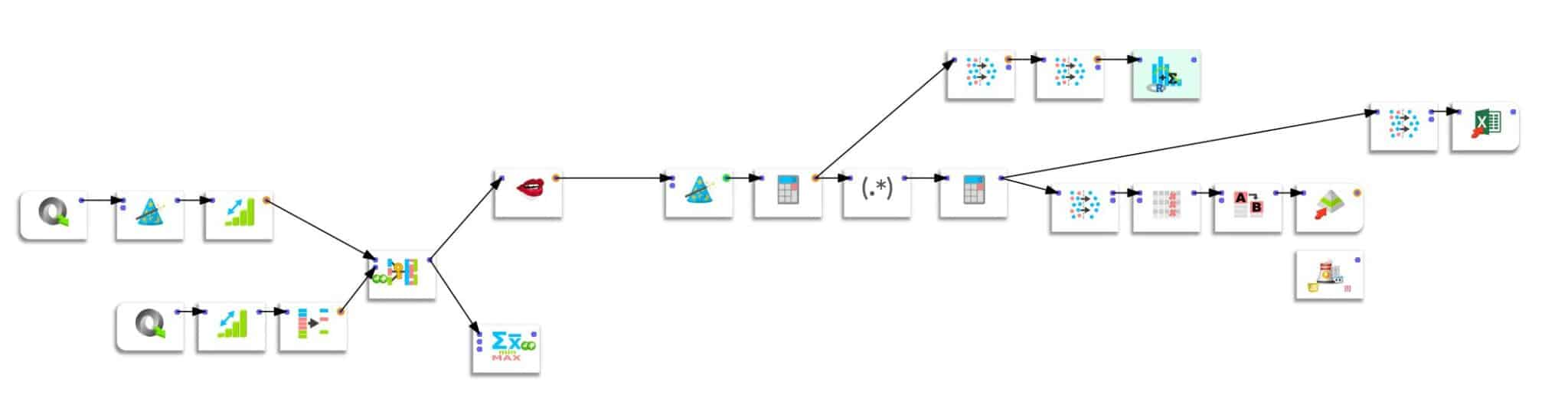Earn money by answering our surveys

# 224 words, the magic number to boost the impact of your LinkedIn posts

Word count is one of the factors that determines the success of a post on LinkedIn, along with hashtags. I have made an analysis of nearly 5 million posts in 100 countries that shows a linear correlation between the word count of a post on LinkedIn and its number of likes/comments. Posts between 224 and 227 words are 6x more likely to get more than 100 reactions than the average. If you’re interested in this topic, subscribe to my newsletter below, and don’t forget to send me a connection request… on LinkedIn, of course 🙂.

## The results of the research, at a glance

The analysis results show that the posts containing 224 and 227 words have 5.80 times more probability of reaching 100 likes/comments than the average.

The data of 4.599 million LinkedIn posts were provided by Linkalyze and analyzed with the Timi suite (Anatella for the data preparation and Modeller for the modeling).

## Why does the word count increase the reach on LinkedIn?

Since LinkedIn’s algorithm emphasizes “dwell time,” the more time spent reading a post, the more it will be recommended by the algorithm.

Logically, the number of words should influence the reading time … and thus the promotion of the post by the algorithm.

## The results in detail

You can see the detailed results on the graph below (produced with Modeller from Timi). A few words of explanation are necessary to understand it properly.

First of all, I eliminated the outliers by removing all accounts with more than 25,000 subscribers. Then, I defined a binary target for the modeling exercise: the probability of getting 100 reactions (likes + comments). This only happened to me once, so I know it’s a complicated feat.

Let’s move on to the graph.The number of words contained in the analyzed LinkedIn posts are indicated on the x-axis:

• 50% of the posts on LinkedIn contain less than 34 words
• 80% of the posts on LinkedIn contain less than 86 words
• 90% of the posts on LinkedIn contain less than 132 words

On the ordinate, on the left, are indicated the number of observations according to the number of words. For example, there were 392952 posts containing 1 or 2 words and 130364 containing 3 or 4 words.

The gray line represents the average number of posts in the sample that received more than 100 reactions (Likes + comments).

Finally, the orange curve (x-axis on the right) represents the percentage of posts with more than 100 reactions as a function of the number of words.

First of all, we observe that beyond 54 words (intersection of the orange curve with the grey line), the reach of the LinkedIn post exceeds the average. The first conclusion is that you should always compose LinkedIn posts of more than 54 words to increase the post’s visibility.

We also observe that the orange curve has 2 parts:

• Up to 176 words, the probability increases by 0.05% with each word added
• Beyond 176 words, the probability increases by 0.18% with each word added

In conclusion, for posts of more than 176 words, the probability of reaching 100 likes/comments increases 3.52x faster than with a post of fewer than 176 words.In one of my first analyses on the impact of the word count on the virality of LinkedIn posts, I estimated the optimum at 216-218 words.

In another research (see graph above), I had already suspected this correlation on a dataset of limited size. At that time, I estimated the ideal size in the number of words at between 216-218.

## Methodology

I have analyzed data from 4.599 million LinkedIn posts with Anatella. Two databases in JSON format were reconciled (see data preparation pipeline below):

• Their authors’ databaseA “Number of reactions” variable was created by adding the number of likes and comments. I had shown in another analysis the number of reactions thus defined was correlated to the number of views.

The statistical modeling was realized using Modeller by including the following variables:

• number of words
• number of hashtags
• number of connections
• country
• language

##crédits : Shutterstock

## Conclusion

This analysis conducted on 4.599 million LinkedIn posts clearly shows that the length of the content influences the visibility (and virality) of a post. Suppose 80% of the posts on LinkedIn contain less than 86 words, and a post has on average only a 3.7% probability of reaching 100 likes/comments. In that case, this probability reaches 20%, with posts containing between 224 and 227 words.

Therefore, it is in your best interest to write lengthy (and interesting, see my 4 golden rules here) posts, as this will send a positive signal to the LinkedIn algorithm.

Tags: , ,Dr. Pierre-Nicolas Schwab is the founder of IntoTheMinds. He specializes in e-commerce, retail and logistics. He is also a research fellow in the marketing department of the Free University of Brussels and acts as a coach for several startups and public organizations. He holds a PhD in Marketing, a MBA in Finance, and a MSc in Chemistry. He can be contacted by email, Linkedin or by phone (+32 486 42 79 42)